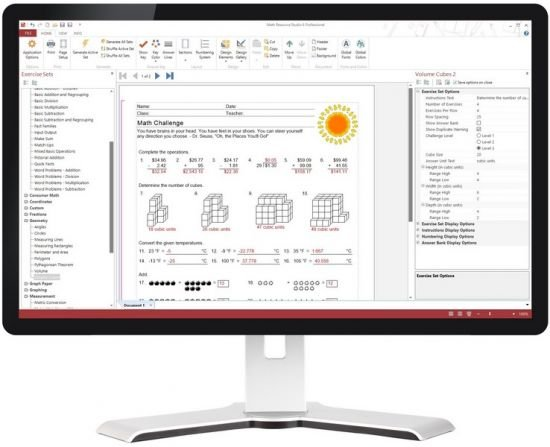Languages: English | File Size: 40.56 MB

Provide students with the precise skills development and math practice they need as part of a differentiated numeracy program. Create individual or class sets of professional math worksheets, workbooks, or tests quickly and effortlessly saving valuable preparation time and resources.

Hundreds of worksheet activity configurations
A total of more than 120 unique math worksheet activities in all. And with the many optional settings for each activity, there are literally hundreds of activity configurations available.

– basic number operations
– number concepts
– algebra
– geometry
– fractions
– numeration
– number lines
– time
– consumer math
– math puzzles
– ratio and percent
– measurement
– tables and drills
– graphing
– coordinates

New math worksheets in version 6:
– Mixed Basic Operations
– Temperature Conversion
– Volume Cubes
– Volume
– Custom Word Problems
– Custom Questions
– Multiplication Word Problems
– Time Conversions
– Sudoku
– Division Word Problems
– Number Lines – Integers
– Number Lines – Decimals
– Number Lines – Fractions
– Plot Lines
– Slope Intercept
– Inequalities

Advanced addition, subtraction, multiplication, multiplication with powers of ten, division, division with remainders, division with no remainders, mixed operations, multiple addends, and multiple operations.

Basic Operations
Basic addition, doubles addition, addition with regrouping control, division, multiplication, subtraction, subtraction with regrouping control, fact families, input output, make sums, match-ups, mixed operations, pictorial addition, quick facts, word problems with addition, subtraction, multiplication, and division.

Fractions
Addition, subtraction, multiplication, and division with fractions, comparing fractions, division with whole numbers, fraction identification with grids and rectangles, fractions and decimals, mixed numbers and improper fractions, mixed operations with fractions, multiplication and division with whole numbers, equivalent fractions, and simplifying fractions.

Algebra
Solving equations with defined variables, equations with single variable, inequalities, number problems, and pre-algebra.

Puzzles
Across-downs, addition box, magic squares, multiplication box, number patterns, secret trails, and sudoku.

Tables and Drills
Circle and table drills for addition, subtraction, multiplication, and division practice, plus counting tables.

Geometry
Angles, circumference and area of circles, measuring lines, measuring rectangles, perimeter and area of rectangles, triangles, parallelograms, and trapezoids, identification of polygons, pythagorean theorem, volume of rectangular and triangular prisms, cylinders, cones, and spheres, and introduction to volume with cubes.

Time
Telling time and time passages with different clock faces in selected increments from 1 minute to 60 minutes, and time conversions: minutes to hours, hours to minutes, etc.

Numeration
Activities for number identification and position, counting, expanded notation, factors and GCF, multiples and LCM, number comparison, ordering numbers, place values, prime numbers, and rounding numbers.

Number Concepts
Exponents, mean, median, mode, and range, roman numerals, roots, and scientific notation.

Measurement
Metric weights and measures, US weights and measures, conversion between metric and US measures, reading thermometers in fahrenheit and celsius, and conversion between fahrenheit and celsius.

Ratio & Percent
Percent of numbers basic and advanced, ratio conversions, and percent and decimals.

Coordinates
Cartesian coordinates in single or four quadrants, plotting lines, and graphing lines in slope intercept form.

Graphing
Activities for working with line and bar graphs.

Consumer Math
Counting money with images of coins or bills (notes), money in words, word problems for compound interest, simple interest, shopping, and wages.

Number Lines
Number lines for placement of integers, decimals, and fractions.

Be more productive
Producing math practice worksheets with Math Resource Studio is as simple as selecting the types of questions you want and how many, setting a few options to control the difficulty of the questions, and clicking the generate button. A powerful random generation engine takes over and creates an original set of questions, calculates the answers, and in just seconds you have a completely unique and original math worksheet.

Easily create the exact math worksheets you require to support your instructional objectives and desired learning outcomes. Math Resource Studio allows you to precisely specify the type and level of worksheet needed to assess prior learning, reinforce lesson concepts, and evaluate what has been learned.

Save time
Never spend valuable and scarce hours preparing math worksheets again. No more picking through file folders of old photocopies and blackline masters, flipping through workbooks, getting lost in the mazes of online worksheet providers, or manually creating one with pencil and paper. The exact differentiated math worksheet activity you require for tomorrow’s lesson will be only seconds away.

RELEASE NOTES:
Fixed screen display after update to Windows 10 caused flickering of application window when resizing.

Requirements: Windows® 10, 8/8.1, 7, Vista, XP

HOMEPAGE
https://www.schoolhousetech.com